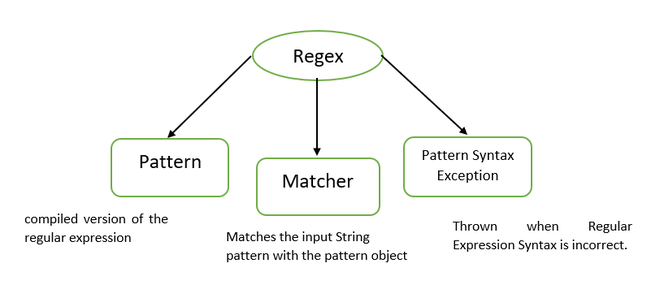Open in App
Not now

# Metacharacters in Java Regex

• Last Updated : 16 Nov, 2021

Regex stands for Regular Expression, which is used to define a pattern for a string. It is used to find the text or to edit the text. Java Regex classes are present in java.util.regex package, which needs to be imported before using any of the methods of regex classes.

java.util.regex package consists of 3 classes:

1. Pattern
2. Matcher
3. PatternSyntaxExceptionClasses in regex package

## Metacharacters

Metacharacters are like short-codes for common matching patterns.

### Usage of Metacharacters

• Precede the metacharacter with backslash (\).

## Java

 `// Java program to demonstrate the``// Digit & Non Digit related Metacharacters`` ` `import` `java.io.*;``import` `java.util.regex.*;``class` `GFG {``    ``public` `static` `void` `main(String[] args)``    ``{``        ``// \d represents a digit``        ``// represents a number so return true``        ``System.out.println(Pattern.matches(``"\\d"``, ``"2"``)); ``//true`` ` `        ``// Comparing a number with character so return false``        ``System.out.println(Pattern.matches(``"\\d"``, ``"a"``)); ``//false`` ` `        ``// \D represents non digits``        ``// Comparing a non digit with character so return``        ``// true``        ``System.out.println(Pattern.matches(``"\\D"``, ``"a"``)); ``//true`` ` `        ``// comparing a non digit with a digit so return``        ``// false``        ``System.out.println(Pattern.matches(``"\\D"``, ``"2"``)); ``//false``    ``}``}`

Output

```true
false
true
false```

Explanation

• d metacharacter represents a digit from 0 to 9. So when we compare “d” within the range, it then returns true. Else return false.
• D metacharacter represents a non-digit that accepts anything except numbers. So when we compare “D” with any number, it returns false. Else True.

## Java

 `// Java program to demonstrate the``// Whitespace and Non-Whitespace Metacharacters`` ` `import` `java.io.*;``import` `java.util.regex.*;``class` `GFG {``    ``public` `static` `void` `main(String[] args)``    ``{``        ``// comparing any whitespace character with a white``        ``// space so return true else false``        ``System.out.println(Pattern.matches(``"\\s"``, ``" "``)); ``//true``        ``System.out.println(Pattern.matches(``"\\s"``, ``"2"``)); ``//false``       ` `        ``// comparing any non whitespace character with a non``        ``// white space character so return true else false``        ``System.out.println(Pattern.matches(``"\\S"``, ``"2"``)); ``//true``        ``System.out.println(Pattern.matches(``"\\S"``, ``" "``)); ``//false``    ``}``}`

Output

```true
false
true
false```

Explanation

• s represents whitespace characters like space, tab space, newline, etc. So when we compare “s” with whitespace characters, it returns true. Else false.
• S represents a Non-whitespace character that accepts everything except whitespace, So when we compare “S” with whitespace characters, it returns false. Else true

## Java

 `// Java program to demonstrate the``// Word & Non Word Metacharacters`` ` `import` `java.io.*;``import` `java.util.regex.*;`` ` `class` `GFG {``    ``public` `static` `void` `main(String[] args)``    ``{``        ``// comparing any word character with a word``        ``// character so return true else false``        ``System.out.println(Pattern.matches(``"\\w"``, ``"a"``)); ``//true``        ``System.out.println(Pattern.matches(``"\\w"``, ``"2"``)); ``//true``        ``System.out.println(Pattern.matches(``"\\w"``, ``"\$"``)); ``//false`` ` `        ``// comparing any non word character with special``        ``// symbols & whitespaces return true else false``        ``System.out.println(Pattern.matches(``"\\W"``, ``"2"``)); ``//false``        ``System.out.println(Pattern.matches(``"\\W"``, ``" "``)); ``//true``        ``System.out.println(Pattern.matches(``"\\W"``, ``"\$"``)); ``//true``    ``}``}`

Output

```true
true
false
false
true
true```

Explanation

• w represents word character which accepts alphabets (Capital & small) and digits [0-9]. So when we compare “wwith an alphabet or number returns true. Else false.
• W represents a non-word character that accepts anything except alphabets and digits. So when we compare “Wwith an alphabet or number returns false. Else true.

## Java

 `// Java program to demonstrate the``// Word & Non Word Boundary Metacharacters`` ` `import` `java.io.*;``import` `java.util.regex.*;``class` `GFG {``    ``public` `static` `void` `main(String[] args)``    ``{``        ``// \b says that a string must have boundary letters``        ``// of word characters``        ``System.out.println(``            ``Pattern.matches(``"\\bGFG\\b"``, ``"GFG"``)); ``// true``        ``System.out.println(``            ``Pattern.matches(``"\\b@GFG\\b"``, ``"@GFG"``)); ``// false`` ` `        ``// \B says that a string must have non word``        ``// characters as boundaries``        ``System.out.println(Pattern.matches(``            ``"\\B@GFG@\\B"``, ``"@GFG@"``)); ``// true``        ``System.out.println(``            ``Pattern.matches(``"\\BGFG\\B"``, ``"GFG"``)); ``// false``    ``}``}`

Output

```true
false
true
false```

Explanation:

• b indicates a string must have boundary elements of word characters, i.e., either digits or alphabets. So here, the GFG string has boundaries G, G, which are word characters so returns true. For the @GFG string, the boundary elements are @, G where @ is not word character, so return false.
• B indicates a string must have boundary elements of Non-word characters, i.e., it may have anything except digits or alphabets. So here @GFG@ string has boundaries @,@ which are Non-word characters so returns true. For the GFG string, the boundary elements are G, G, which are word characters, returning false.

Example:

## Java

 `// Java program to demonstrate all the ``// Metacharacters in the Java Regex`` ` `import` `java.io.*;``import` `java.util.regex.*;`` ` `class` `GFG {``    ``public` `static` `void` `main(String[] args)``    ``{``        ``// \d-number``        ``// \D-Non Digit``        ``// \s-Any White Space``        ``// \S-Non White Space character``        ``// \w-any word character like  numbers/characters``        ``// \W-special symbols``        ``System.out.println(Pattern.matches(``            ``"\\d\\D\\s\\S\\w\\W"``, ``"1G FG!"``)); ``// true``       ` `        ``System.out.println(Pattern.matches(``            ``"\\d\\D\\s\\S\\w\\W"``, ``"Geeks!"``)); ``// false``    ``}``}`

Output

```true
false```

My Personal Notes arrow_drop_up